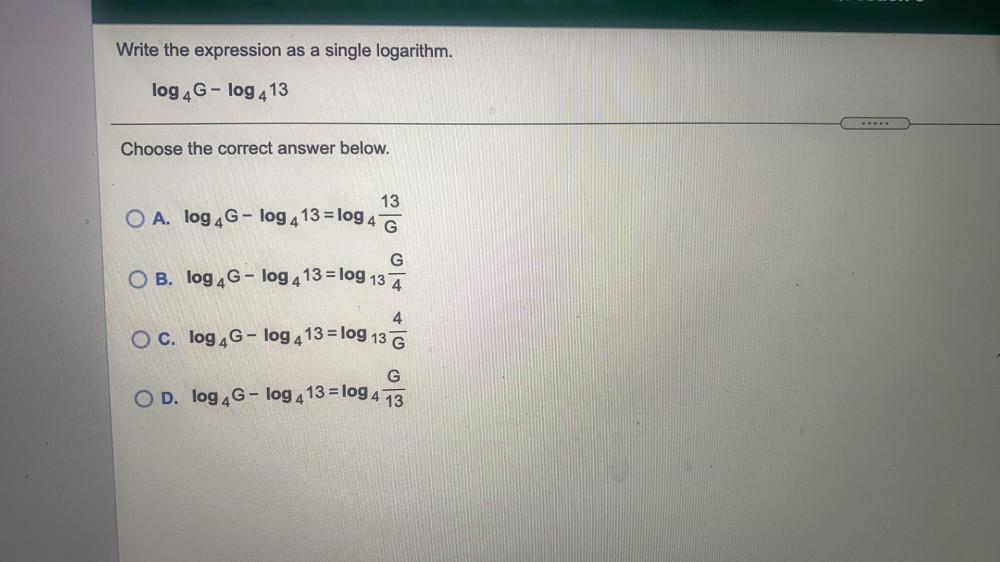Question:

# Write the expression as a single logarithm. log 4G-log 413 Choose the correct answer below. 13 O A. log 4G - log 4 13 = log 4 GWrite the expression as a single logarithm. log 4G-log 413 Choose the correct answer below. 13 O A. log 4G - log 4 13 = log 4 G G O B. log 4G - log 4 13 = log 13 Ā 4 O c. log 4G - log 4 13 = log 13 G G O D. log 4G - log 4 13 = log 4 13